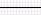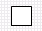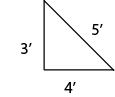First Line Sales
PO Box 200
Vernon, VT 05354

www.firstlinesales.com

Construction Estimating Formulas & Useful Information

Formulas, equations, tables, facts, specifications and other
information useful in planning and estimating construction,
decorating and other projects.

Three dimensions of estimating:Linear MeasureSquare MeasureCubic Measure 12 inches = 1 lineal ft. 12" x 12" = 144sq" = 1sq ft. 12" x 12" x 12" = 1728 cu" = 1 cubic ft. 3 lineal feet = 1 yard. 3' x 3' = 9sq feet = 1sq yd. 3' x 3' x 3' = 27 cu feet = 1 cubic yard. 5,280 lin ft = 1 mile. 43,560sq' = 1 acre. 36" x 36" x 36" = 46,656 cu" = 1 cubic yard. Inches x .0833 = ft. Inches / 12 = ft. Sq" x .00694 = sq ft. Cu" x .0005787 = cu'. Inches x .2778 = yds. Sq" x .007716 = sq yds. Cu' x .0002143 = cu yds. Feet x .3333 = yds. Sq' x .11111 = sq yds. Cu' x .03704 = cu yds. 100sq feet = 1 square (measure used in roofing).

Useful Mathematical Formulas:

1. One of the most useful formulas is the Pythagorean theorem.  The theorem states that the square of the hypotenuse of a right (90 degree angle) triangle is equal to the sum of the squares of its legs.

For Example:        (3' x 3' = 9') + (4' x 4' =16') = 25',  √25=5This can be used for a wide variety of uses from squaring up a concrete form for a foundation, to attaching a new addition squarely to an existing building, or measuring a countertop -anywhere you must be certain of a right angle.  Just plug in your numbers and get a calculator that has a square root function.

This information is believed to be accurate.  The Polymer Roof Factory or First Line Sales will not be liable for errors and omissions.  Specifications, detail, and other information should be verified by the user.